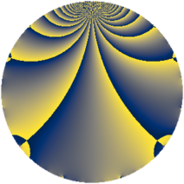# Properties

 Label 3549.2.gLevel $3549$ Weight $2$ Character orbit 3549.g Rep. character $\chi_{3549}(3548,\cdot)$ Character field $\Q$ Dimension $392$ Sturm bound $970$

# Related objects

## Defining parameters

 Level: $$N$$ $$=$$ $$3549 = 3 \cdot 7 \cdot 13^{2}$$ Weight: $$k$$ $$=$$ $$2$$ Character orbit: $$[\chi]$$ $$=$$ 3549.g (of order $$2$$ and degree $$1$$) Character conductor: $$\operatorname{cond}(\chi)$$ $$=$$ $$273$$ Character field: $$\Q$$ Sturm bound: $$970$$

## Dimensions

The following table gives the dimensions of various subspaces of $$M_{2}(3549, [\chi])$$.

Total New Old
Modular forms 512 432 80
Cusp forms 456 392 64
Eisenstein series 56 40 16

## Trace form

 $$392q + 384q^{4} + O(q^{10})$$ $$392q + 384q^{4} + 368q^{16} - 312q^{25} - 52q^{30} + 52q^{36} + 52q^{42} - 48q^{43} + 36q^{49} + 4q^{51} + 336q^{64} - 136q^{79} + 32q^{81} + 96q^{88} + O(q^{100})$$

## Decomposition of $$S_{2}^{\mathrm{new}}(3549, [\chi])$$ into newform subspaces

The newforms in this space have not yet been added to the LMFDB.

## Decomposition of $$S_{2}^{\mathrm{old}}(3549, [\chi])$$ into lower level spaces

$$S_{2}^{\mathrm{old}}(3549, [\chi]) \cong$$ $$S_{2}^{\mathrm{new}}(273, [\chi])$$$$^{\oplus 2}$$